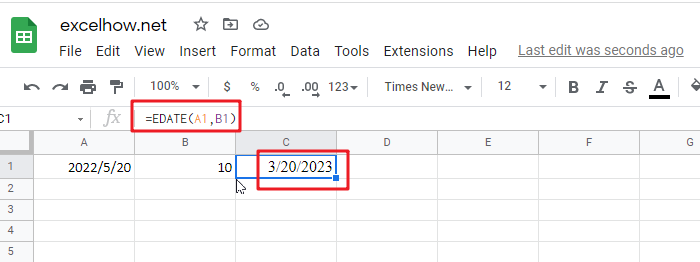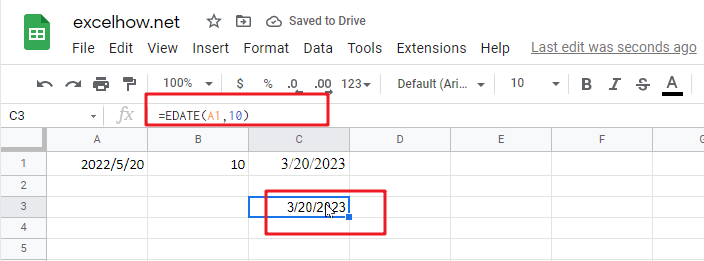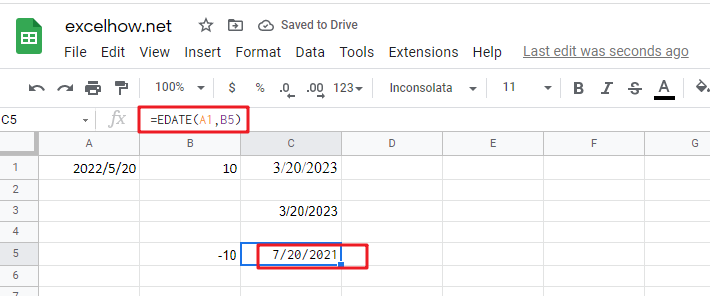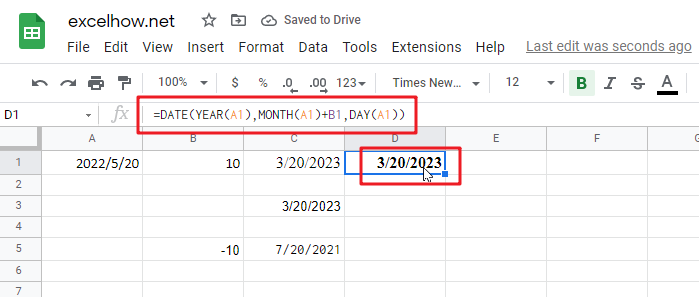It is important to adjust time periods when performing financial modeling. In Google Sheets, you can use the MONTH function to add a specific number of months to a date. The MONTH function takes one argument: the number of months you want to add. Here will show you how to add months to date in Google Sheets and even provide some examples that might be useful!## Method1: Add Months to Date using EDATE Function

Generic formula:

`=EDATE(Sart_date, months)`

### Arguments

• `Start_date`: the starting date on which you want to add months
• `Months`: the month that you wish to add

### Return Value:

The function will return the date that is the indicated number of months after the start_date. If you enter a negative number of months, it’ll count back from the end date.

When you enter the MONTH function, Google Sheets looks at the date in the cell that you specify as the start_date argument. It then adds the number of months that you entered as the second argument to that date. If you enter a negative number for the second argument, Google Sheets subtracts that number of months from the start_date.

To add a number of months is placed at B2 and add months to the date in cell A2. The formula for this would be:

`=EDATE(A1,B1)`Or simply enter the number you want in your formula, and it will be replaced with that value.

`=EDATE(A1,10)`### Explanation

This will add 10 months to the date in cell A1. The function will return the date that is the indicated number of months after the start_date. If you enter a negative number of months, it’ll count back from the end date.

The EDATE function is a great way to find out your next date. Simply enter any valid date and then select how many months away from now you want the new one! It’s really easy; just feed it with an amount less than or equal to the number of desired days between these two points-and voila.## Method2: Add Months to Date using Date Function

Generic formula:

`=DATE(YEAR(date),MONTH(date))+months,DAY(date)))`

### Arguments

• `Date`: The starting date on which you want to add months.
• `Months`: Months are important when adding or subtracting a certain number of months from/to dates. They add the indicated amount, depending on whether it’s positive and negative values respectively; for example, -1 means one month less than what you want (in other words: ago).
• `YEAR`(date): The year component of the date in Date.
• MONTH(date): The month component of the date in Date.
• `DAY`(date): The day component of the date in Date.

### Return Value:

A date is the indicated number of months after the start_date. If you enter a negative number of months, it’ll count back from the end date.

### How This Formula Work

To add months to the date in cell A1, and a number of added is placed at B1. The formula for this would be as follows:

`=DATE(YEAR(A1),MONTH(A1)+B1,DAY(A1))`With the Year, Month, and Day functions, you can easily create your date from any combination of these three values. With the Date function, you can create dates based on any year and month of your choice.

### Related Functions

The Google Sheets DAY function returns a day of a date (from 1 to 31).The DAY function is a build-in function in Microsoft Excel and it is categorized as a DATE and TIME Function.The syntax of the DAY function is as below:= DAY (date_value)…
The Google Sheets EDATE function returns the serial number that represents the date that is a specified number of months before or after a specified date.The syntax of the EDATE function is as below:=EDATE (start_date, months)…
The Google Sheets DATE function returns the serial number for a date.The syntax of the DATE function is as below:= DATE (year, month, day)…
The Google Sheets MONTH function returns the month of a date represented by a serial number. And the month is an integer number from 1 to 12.The syntax of the MONTH function is as below:=MONTH (serial_number)…
The Google Sheets YEAR function returns a four-digit year from a given date value, the year is returned as an integer ranging from 1900 to 9999. The syntax of the YEAR function is as below:=YEAR (serial_number)…

Related Posts

Find the Closest Data to the Data Provided in Google Sheets

In our daily work, we may encounter such an issue that to find the closest value to a certain value. In fact, google sheets internal functions can help us solve this problem. In today’s article, we will show you how ...

Basic Array Formula With Examples in Google Sheets

Are you searching for an article for getting the basic array formula with different examples for better understanding? Then congratulations because you have just landed on the right article. In this article, you would get to know the basic array ...

Average Of Numbers With Multiple Criteria in Google Sheets

Have you ever come across a task to calculate the average of the numbers with respect to multiple criteria in google sheets? Are you tired of doing this cumbersome task manually? Are you willing to do this task smartly in ...

Calculate Average Of Last 5 Or N Values In Columns in Google Sheets

Suppose you come across a task where you need to calculate the average of the last 2 or 3 numeric values in google sheets, then what would you do? If you are new to google sheets, then your first attempt ...

Find And Retrieve Missing Values in Google Sheets

Find and retrieve is a common basic operation in Google Sheets for tables. In out daily work, we may encounter this situation that some data was lost after several operations on a table in Google Sheets. In fact, we can ...

Calculating Average Of The Numbers in Google Sheets

Are you weary of investing a lot of time and effort in manually calculating the average of the numbers by including or excluding 0 and calculating the average of the top 3 scores? Then congratulations because you have just landed ...

Calculate The Average Of The Last 3, 5, Or N Numeric Values In Google Sheets

As an Google sheets user, you might have come across a task in which you need to calculate the average values of the last 2 numeric values, and you might have done this task manually but suppose if the last ...